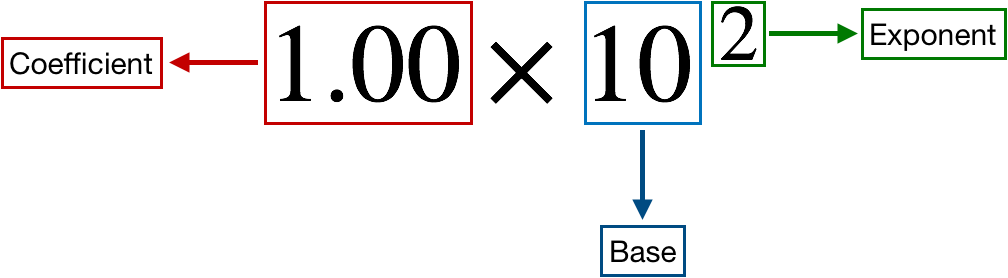# Problem: The diameter of Earth at the equator is 7926.381 mi. Round this number to three significant figures, and express it in standard exponential notation.

###### FREE Expert Solution

We’re being asked to express the diameter of Earth at the equator which is 7926.381 mi in scientific notation rounded off to three significant figures.

Recall that a scientific notation expression is composed of three parts:86% (99 ratings)###### Problem Details

The diameter of Earth at the equator is 7926.381 mi. Round this number to three significant figures, and express it in standard exponential notation.